### Home > PC > Chapter 12 > Lesson 12.2.2 > Problem12-53

12-53.
1. a. Find the images of the four points (0, 0), (1, 0), (1, 1), and (0, 1) by the matrix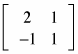. Homework Help ✎1. Graph these points.

2. What is the image of the square whose vertices are the points (0, 0), (1, 0), (1, 1), and (0, 1)? Shade it on the graph you made in part (b).

3. What is the name of this geometric figure?

4. Sketch the images of the lines y = 0, y = 1, x = 0 and x = 1.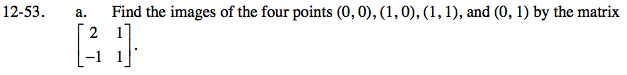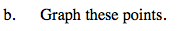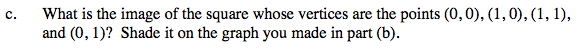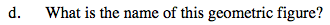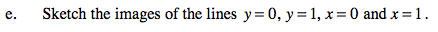For the line y = 1, all points are of the form (a, 1).

$(a,1)\begin{bmatrix}2&1\\-1&1\end{bmatrix}=(2a-1,2a+1)$

Hence x = 2a − 1 and y = a + 1.
Write an equation relating x and y.

$y=\frac{1}{2}x+\frac{3}{2}$

If you graphed the original line and its image, how would it relate to the original square and its image in parts (a) and (b)?